# Ritz and Galerkin Methods

The following article is from The Great Soviet Encyclopedia (1979). It might be outdated or ideologically biased.

## Ritz and Galerkin Methods

widely used direct methods for the solution of, primarily, variational problems and boundary value problems of mathematical analysis (seeBOUNDARY VALUE PROBLEMS and CALCULUS OF VARIATIONS).

Ritz method. The Ritz method is used mostly for the approximate solution of variational problems and of boundary value problems that reduce to variational problems. Let the functional F[y(x)], or a more complicated functional, be defined. Suppose it is required to find a function y(x) such that (1) y(x) takes on the given values α = y(x0) and β = y(x1) at the points x0 and x1 and (2) V[y(x)] reaches an extremum for y(x). The values of the functional involved in the extremum problem, are considered not for all y(x) permissible in the given problem but only for all possible linear combinations of the form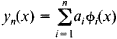with constant coefficients ai made up from the first n functions of some chosen system ɸ1(x), ɸ2(x), …, ɸn(x),…. It should be noted that the effectiveness of the application of the method to the solution of specific problems depends on a successful choice of this system of functions. A necessary condition for the choice of the system of functions ɸi(x) is the requirement that the functions yn(x) satisfy the conditions yn(x0) = α and yn(x1) = β for all values of the parameters ai When such a choice of the yn(x) is made, V[y(x)] becomes a function Φ(a1, a2, …, an) of the coefficients ai. The coefficients are selected so that this function reaches an extremum—that is, the coefficients are determined from the system of equations

δΦ/δai = 0 i = 1, 2,…, n

For example, suppose it is required to solve the problem of the minimum of the integral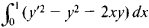under the condition that y(0) = y(1) = 0. We may take xi(1 - x) as the functions ɸi(x); then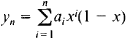If n= 2, then y2 = x(1 - x)(a1 + a2x). To determine the coefficients a1 and a2, we obtain, after calculations, the two equations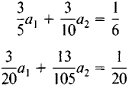The numbers a1 = 71/369 and a2 = 7/41 are the solution of these equations. Consequently,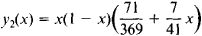The approximate solution obtained differs from the exact solution by a quantity of the order of 0.001.

Under certain conditions concerning primarily the completeness of the system of functions ɸi(x), the approximate solution yn((x) found by this method for the variational problem approaches the exact solution y(x) as n → ∞.

The method was proposed in 1908 by the German mathematician W. Ritz. A theoretical substantiation of the method was given by the Soviet mathematician N. M. Krylov in 1918.

Galerkin method. The Galerkin method is a broad generalization of the Ritz method and is used primarily for the approximate solution of variational and boundary value problems, including problems that do not reduce to variational problems. The basic idea behind the Galerkin method is as follows. Suppose it is required to find a solution, defined in some domain D, of the differential equation

(1) L[u] = 0

(here, L is a differential operator, for example, an operator in two variables) where the solution satisfies at the boundary S of D the homogeneous boundary conditions

(2) u= 0

If the function u is a solution of equation (1) in D, then L[u] is identically equal to zero in this domain and, consequently, is orthogonal to any function in D. The approximate solution of equation (1) is sought in the form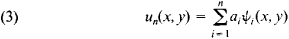where Ψi(x,y) (i = 1, 2, …, n) are linearly independent functions that satisfy the boundary conditions (2) and are the first n functions of some system of functions Ψ1, (x, y), Ψ2 (x, y), …, Ψn(x, y), … that is complete in the given domain. The constant coefficients ai are chosen so that L[un] is orthogonal in D to the first n functions of the system Ψi(x,y):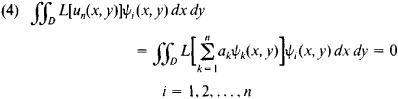For example, suppose it is required to solve in D the Poisson equation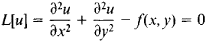under the condition that u = 0 at S. We choose a system of functions ψi(x, y) and seek the solution in the form of (3). The system of equations (4) for determining the coefficients ai has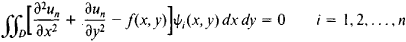In particular, the functions Ψi(x, y) may be chosen with the aid of the following considerations. Let ω(x, y) be a continuous function that has within D continuous second-order partial derivatives. Suppose ω(x, y) > 0 within D and ω(x, y) = 0 at S. Then a system composed of the products of ω (x,y) and different powers of x and y may be chosen as the system of functions Ψi(x, y): Ψ0 = ω, Ψ1 = ωx, Ψ2 = ωx2, Ψ3 = ωxy, …. For example, if a circle S of radius R with its center at the origin is the boundary of D, then we may put ω(x,y) = R2 - x2 - y2.

The Galerkin method is used in solving a broad class of problems. Its most general formulation is given in terms of functional analysis for the solution of equations of the form Au - f = 0, where A is a linear operator defined on a linear space that is dense in some Hilbert space H, u is the unknown, and f is a specified element of H.

The method came into use after it was described in a paper by B. G. Galerkin in 1915. It has been used previously, in 1913, by I. G. Bubnov to solve specific problems of the theory of elasticity. For this reason, it is sometimes called the Bubnov-Galerkin method. A theoretical substantiation of the method was contributed by M. V. Keldysh in 1942.

### REFERENCES

Galerkin, B. G. “Sterzhni i plastinki: Riady v nekotorykh voprosakh uprugogo ravnovesiia sterzhnei i plastinok.” Vestnik inzhenerov, 1915, vol. 1, no. 19, pp. 897-908.
Mikhlin, S. G. Variatsionnye metody v matematicheskoi fizike, 2nd ed. Moscow-Leningrad, 1970.
Kantorovich, L. V., and V. I. Krylov. Priblizhennye metody vysshego analiza, 5th ed. Leningrad-Moscow, 1962.
Ritz, W. “Neue Methode zur Lösung gewisser Randwertaufgaben.” Gesellschaft der Wissenschaften zu Göttingen: Math.-physik. Klasse: Nachrichten. Göttingen, 1908.
Ritz, W. “Über eine neue Methode zur Lösung gewisser Variationsprobleme der mathematischen Physik.” Journal für die reine und angewandte Mathematik. 1909, vol. 135.

V. G. KARMANOV